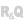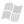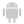Home |Sign in | EnglishHelpEditorsControls (window/page/report)ControlsRange Slider controlHandling a Range Slider by programmingOverviewRetrieving the characteristics of the Range Slider controlModifying the value of the Range Slider controlProperties specific to the management of a Range Slider controlExample of code for using a Range Slider control to zoom on an imageSee alsoEvents associated with a Range Slider controlHandling a Range Slider by programmingProperties associated with the Range Slider controlThe Range Slider control
• Overview
• Retrieving the characteristics of the Range Slider control
• Characteristics that can be retrieved by programming
• Value of the Range Slider control
• Finding out the bounds of the Range Slider control
• Finding out the total range and the visible range of the Range Slider control
• Modifying the value of the Range Slider control
• Properties specific to the management of a Range Slider control
• Example of code for using a Range Slider control to zoom on an image
WINDEVWEBDEVWINDEV MobileOthersHandling a Range Slider by programming
Overview
WINDEV allows you to handle a Range Slider control by programming. To do so, use the variable of the Range Slider control in the code.
The variable of the Range Slider control:
• corresponds to the name of the Range Slider control.
• is initialized with the value of the Range Slider control.
Retrieving the characteristics of the Range Slider control

Characteristics that can be retrieved by programming

The characteristics that can be retrieved by programming are as follows:

Value of the Range Slider control

The value of the Range Slider control corresponds to:
• the position of the left extremity of the box of an horizontal Range Slider control.
• the position of the top extremity of the box of a vertical Range Slider control.
To retrieve the value of the Range Slider control, use the following syntax:
<Position> = <Name of Range Slider>
<Position> is an integer variable.
Remark: You also have the ability to use ..Value.

Finding out the bounds of the Range Slider control

..MaxValue and ..MinValue are used to find out the upper and lower bounds of the Range Slider control.
The lower bound corresponds to the value of the extremity of the Range Slider control:
• left extremity for an horizontal Range Slider control
• top extremity for a vertical Range Slider control.
The upper bound corresponds to the maximum value that can be taken by the Range Slider control with the current configuration of the box of the Range Slider control.
Example:In this example, in position 1:
• the value of the Range Slider control is 21.
• the lower bound is 1.
• the upper bound is 40. This upper bound corresponds to the value of the Range Slider control when the box of the Range Slider control is positioned at the maximum extremity. This case corresponds to position 2. This bound corresponds to the following calculation: Lower bound + (Total range - Visible range).

Finding out the total range and the visible range of the Range Slider control

..TotalRange and ..VisibleRange are used to find out the total range and the visible range of the Range Slider control.
Modifying the value of the Range Slider control
To modify the value of the Range Slider control, use the following syntax:
<Name of Range Slider> = <Value>
<Value> is a value included between the minimum value and the maximum value of the Range Slider control. These values have been described in the description window of the control or by programming, with ..MinValue and ..MaxValue (the upper bound depending on the visible range and on the total range).
For example, for a Range Slider control with the following charateristics:
• LowerBound = 1
• TotalRange = 5
• VisibleRange = 2
The upper bound will be 4 (1 + 5 -2)
The possible values will be 1, 2, 3 and 4
Remark: You also have the ability to use ..Value to modify the value of the Range Slider control.
Properties specific to the management of a Range Slider control
The following properties are used to manage the characteristics of a Range Slider control by programming.
 Scroll Used to find out and modify the value of the scroll step for a Range Slider control. ScrollValue Used to find out and modify the value of the increment step for a Range Slider control. MaxValue Used to find out and modify the upper bound of a Range Slider control. MinValue Used to find out and modify the lower bound of a Range Slider control.Versions 15 and laterTotalRangeNew in version 15TotalRangeTotalRange Used to find out and modify the total number of data represented by the Range Slider control.Versions 15 and laterVisibleRangeNew in version 15VisibleRangeVisibleRange Used to find out and modify the width of the scrollbar box in a Range Slider control.
To find out the entire list of WLanguage properties that can be used with a Range Slider control, see Properties associated with the Range Slider control.
Example of code for using a Range Slider control to zoom on an image
The following code is called whenever a Range Slider control is modified and it used to perform a zoom on an image area defined by the visible range of the Range Slider control.
PROCEDURE DisplayImage()

// Copy the area corresponding to the zoom bar of the two Range Slider controls
// (1 horizontal and 1 vertical) in the entire destination Image control
dCopyBlt(IMG_SOURCE, IMG_DESTINATION, copySrcCopy, ... // "Direct" copy
// Lower bound of Range Slider
RGS_HORIZONTAL..Value, RGS_VERTICAL..Value, ...
// The entire width of the zoom area
RGS_VERTICAL..VisibleRange, RGS_HORIZONTAL..VisibleRange, ...
// Copy into the destination control at the top left
1, 1, ...
// Copy into the destination control on the entire surface
IMG_DESTINATION..Height, IMG_DESTINATION..Width)
Minimum version required
• Version 15